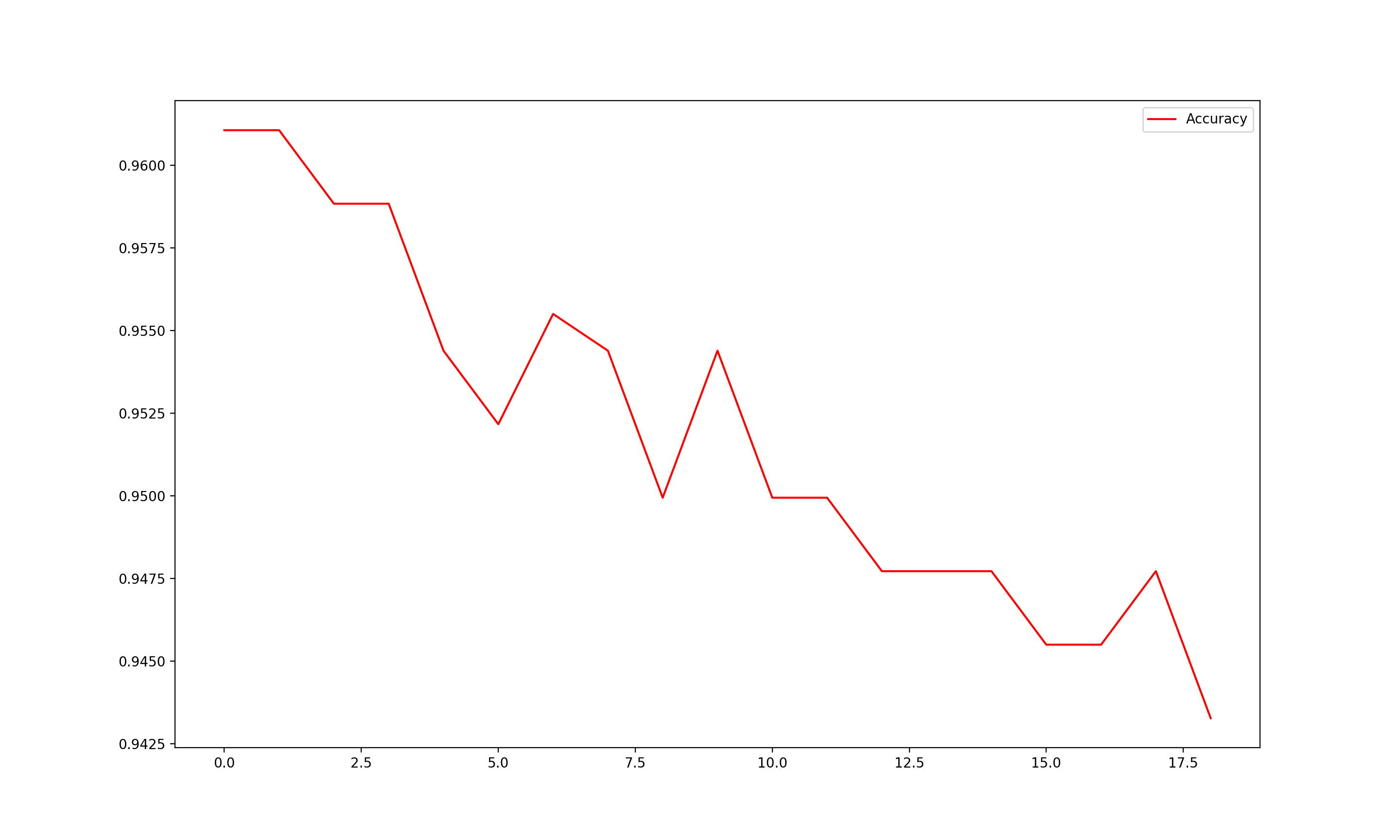Jiahonzheng's Blog

# ML KNN

2019/09/01 ShareKNN is a nonparametric method used for classification and regression. It is a type of instance-based learning, or lazy learning, where the function is only approximated locally and all computation is differed until classification.

## How does it work?We use an example from Wikipedia to explain the decision-making process of KNN: The test sample (green dot) should be classified either to blue squares or to red triangles. If k = 3 (solid circle) it is assigned to the red triangles because there are 2 triangles and only 1 square inside the inner circle. If k = 5 (dashed line circle) it is assigned to the blue squares (3 squared vs. 2 triangles inside the outer circle).

So as we can see, KNN is based on feature similarity.

The general decision-making process of KNN is:

• Calculate the distance between the query-instance and all the training samples.

• Sort the distance.

• Determine the nearest neighbors based on the K-th minimum distance.

• Use simple majority of the category of the nearest neighbors as the prediction value of the query instance.

## Show me the code!

We apply the KNN algorithm in the hand written single digit classification problem, where each input sample is an $8 \times 8$ image. Half of them are used as training data and others are used for testing. And we use different $K$ as hyper-parameter to compare their prediction performances.As we can see from the above figure, the choice of $K$ has a great impact on the accuracy.

## Optimization

There are some fast adaptations of the original KNN algorithm, to name a few: